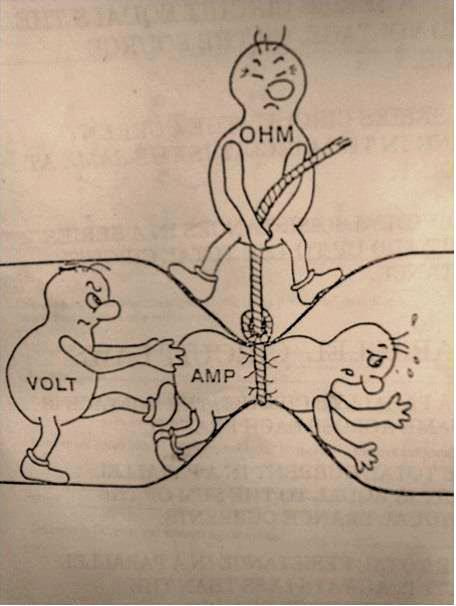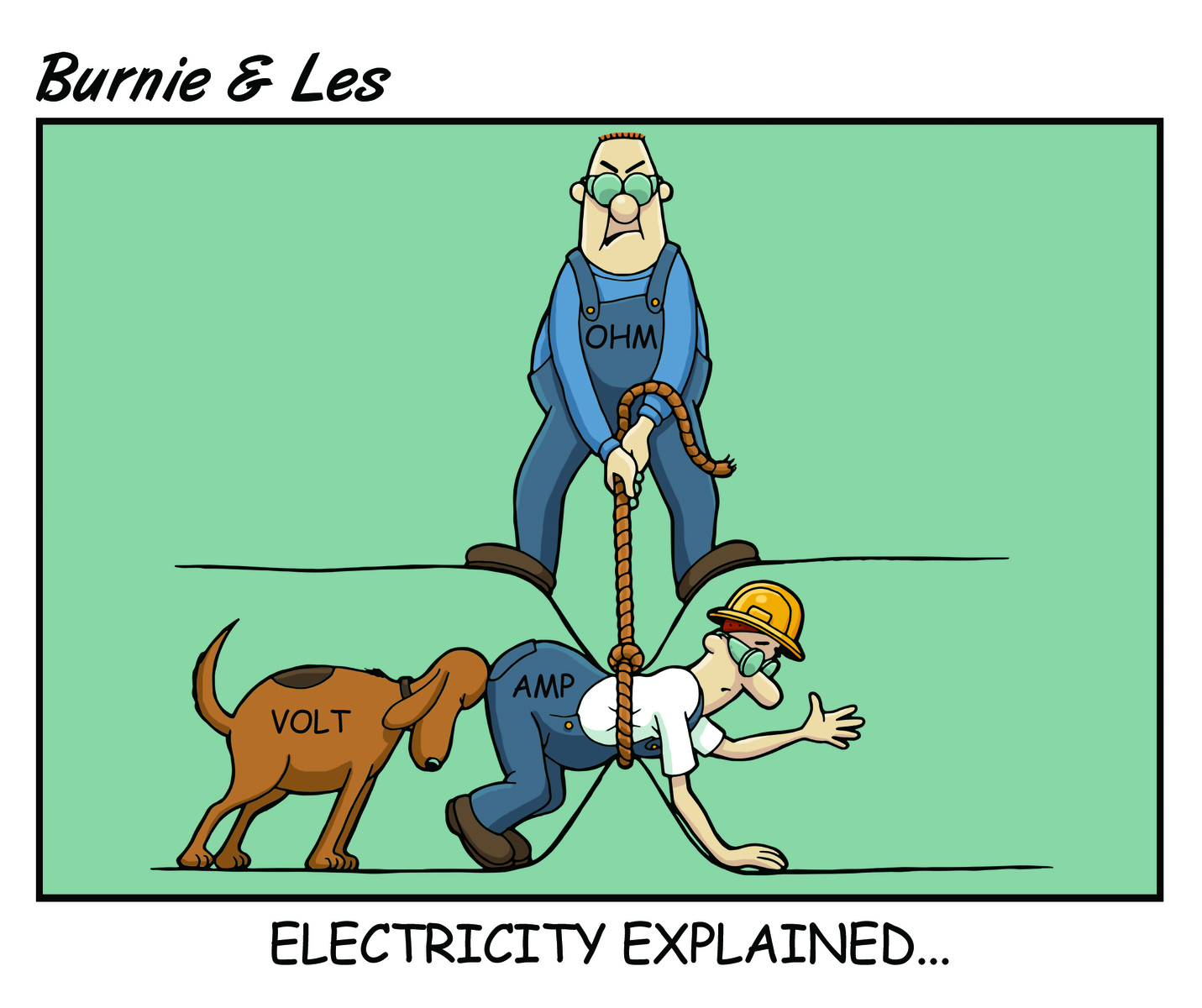## Explain Like I'm FiveOne of the coolest things to come from the internet is the popular term, "Explain Like I'm Five (ELI5)" where a discussion about anything can be going on and someone will comment in a thread saying, "I don't understand this; explain it to me like I'm a five-year-old." Which is pretty much what I asked about electricity and electrical safety devices when I started working here because I have a plumbing background!

I believe it was either Phil or Drew, but they described it for me using plumbing as an analogy. I found a similar analogy of electricity on the internet and an image that inspired us to make a Burnie & Les comic of Electricity Explained. Check it out:Imagine water flowing through a pipe. In our analogy electrons flow through a wire similar to water flowing through a pipe. It's not exactly the same in real life, but it's similar in a lot of ways and will help to explain.

A coulomb is a unit measuring the amount of electrical "charge". The analogy to water breaks down a little with this, but you can think of charge as being a little bit like mass. It's how much "stuff" there is, except mass is the "stuff" that matters for gravity and charge is the "stuff" that matters for electricity.

Current is the flow rate through the pipe. It's how much water (electrons) flow through a certain cross section of the pipe in a given amount of time. In electricity we use the unit Amperes to measure current. The unit of Amperes is equivalent to coulombs/second.

Voltage is like the pressure of the water in the pipe. It's how "forceful" the current pushes through the pipe.

Resistance is a bit like friction from the pipe itself. It's how hard it is to push water (electrons) through the pipe.

An important equation relating these quantities is Ohm's Law, which states Voltage = Current * Resistance. This mathematical relation describes the behavior of electricity in simple circuits and is very fundamental to the physics of electrical circuits. In English you can think of this as meaning "the pressure in the pipe is proportional to the rate of flow times the friction of the pipe".

Watts is actually not directly related to electricity. that is a unit of what is called "power" in physics, which is a way of measuring energy expended over time. Electrical currents are capable of doing work so many electrical devices have a Wattage number listed to explain how much energy the device uses to do its work.

AC means alternating current. DC means direct current. these both refer to methods of transmitting electricity over a distance. DC is almost exactly the same as the way you typically think of water flowing through a pipe. AC transmits by waves instead. Imagine a bathtub full of water. You dunk your hand at one end of the bathtub and it sends a wave of water traveling across the tub until it reaches the other sides, where it does some work at a distant device. That is what alternating current is like (sort of).Standard drives technology developments that include risk-reduction tools and strategies for improved workplace safety and productivity.

By Phil Allen, President of Grace Engineered Products, Inc.connect with us

Hit the button below to win prizes and have a great week!